# The History-State Value Matrix

Understanding the relationship between history values and state values.

This blog discuss the bias seen in A Deeper Understanding of State-Based Critics in Multi-Agent Reinforcement Learning.

The history-state values $Q(h,s,a)$ are defined as the expected return given the agent in state $s$, with history $h$ and taking an $a$. If we only consider one action particular action (say $a_o$), we get a matrix of values that correspond to different states and histories .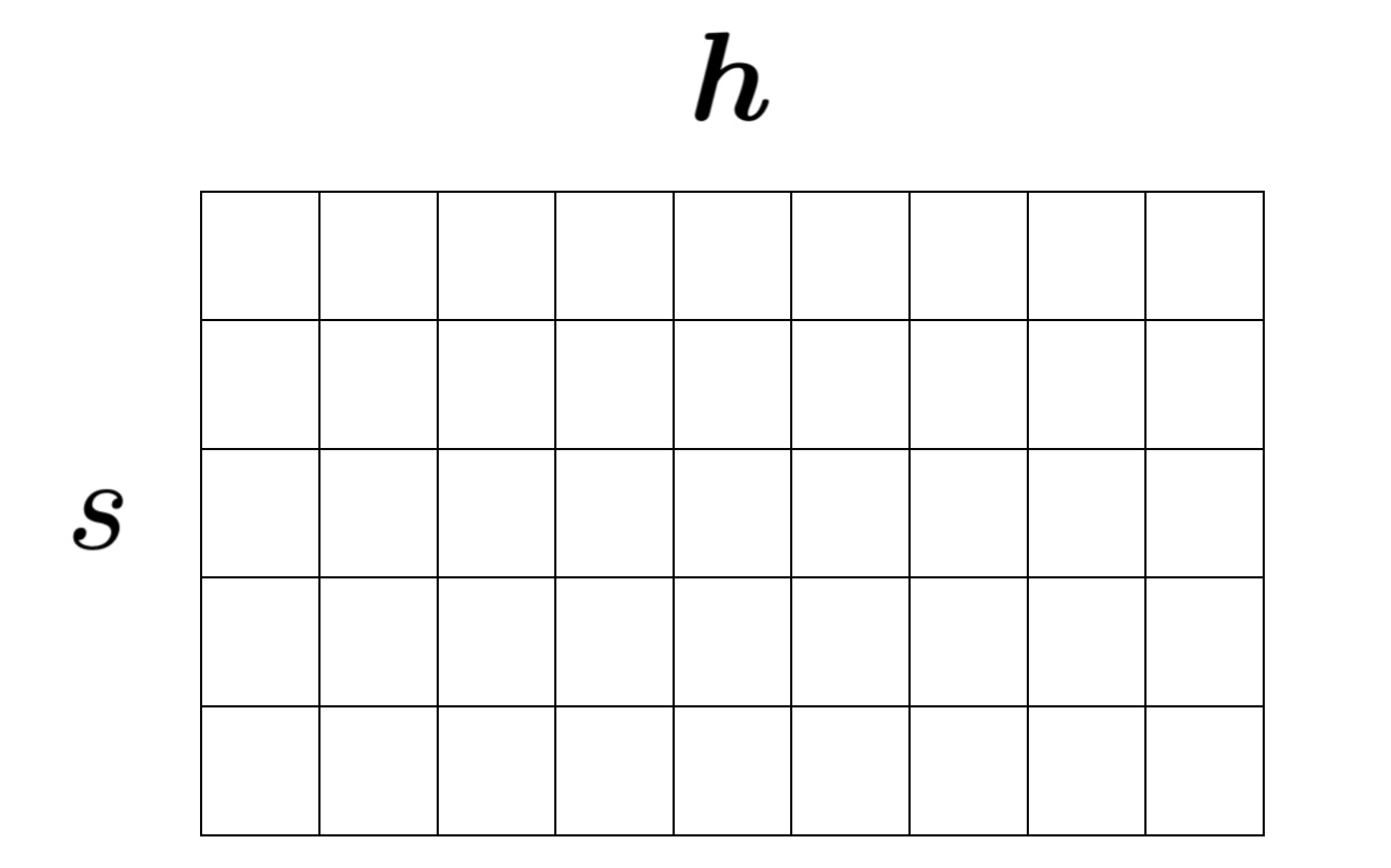When we consider the expected value for a certain history or a certain state, we are implicitly taking an average over a certain column or a certain row respectively: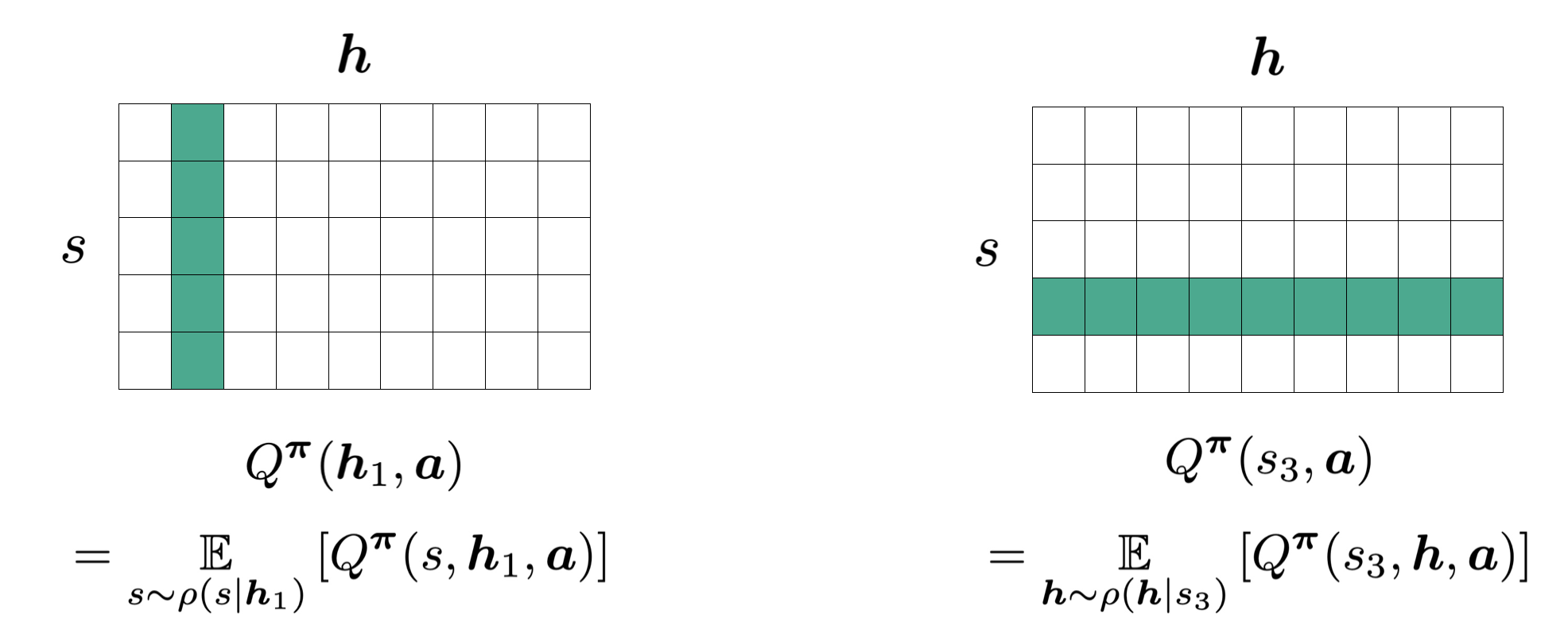If we are to use state values to approximate history values in the fashion seen in state-based critics nowadays, say $\boldsymbol{h}_1$, we should be expecting to use the values from one single column as shown: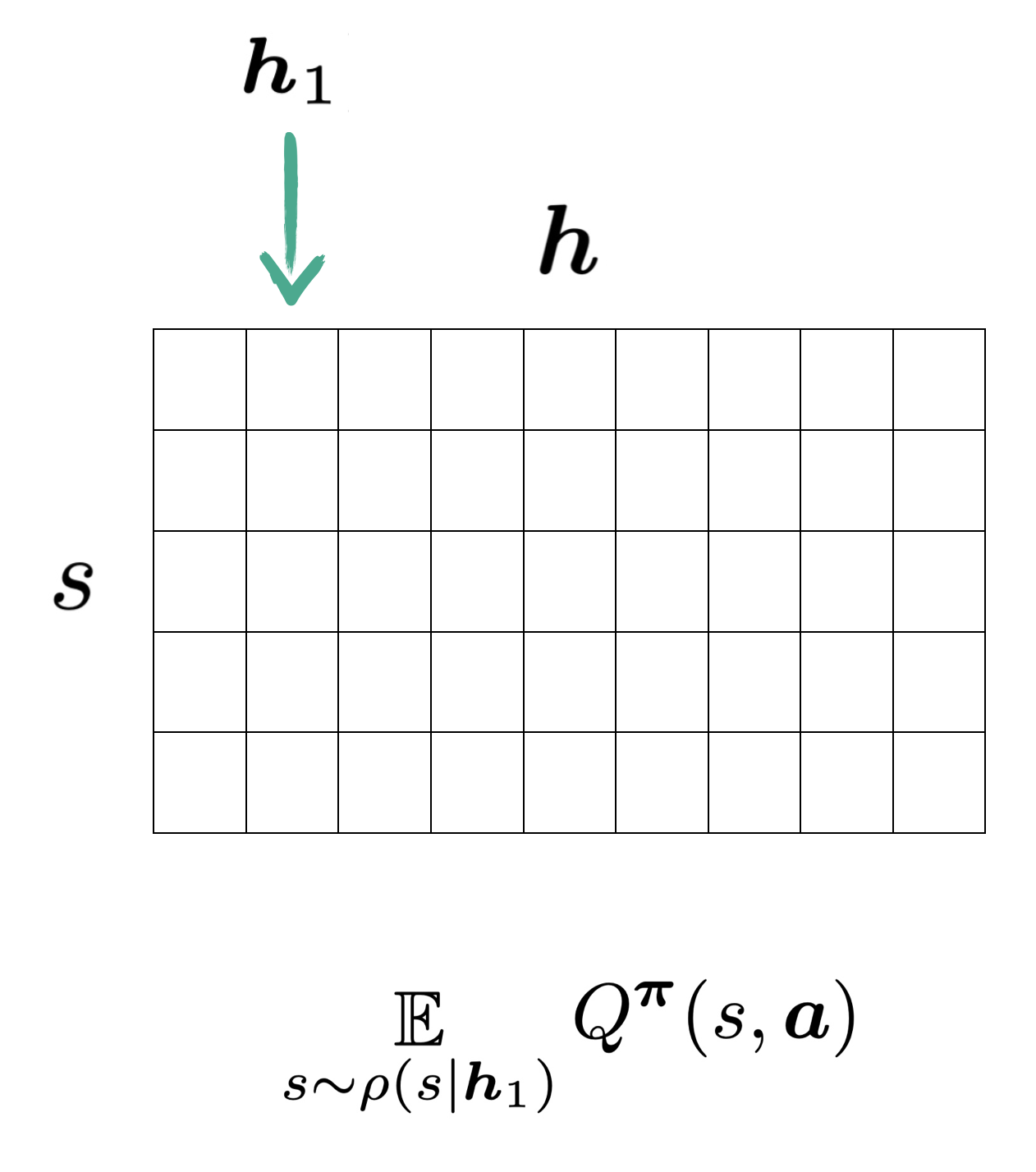The catch, however, is that the state values being used here are themselves averages from entires rows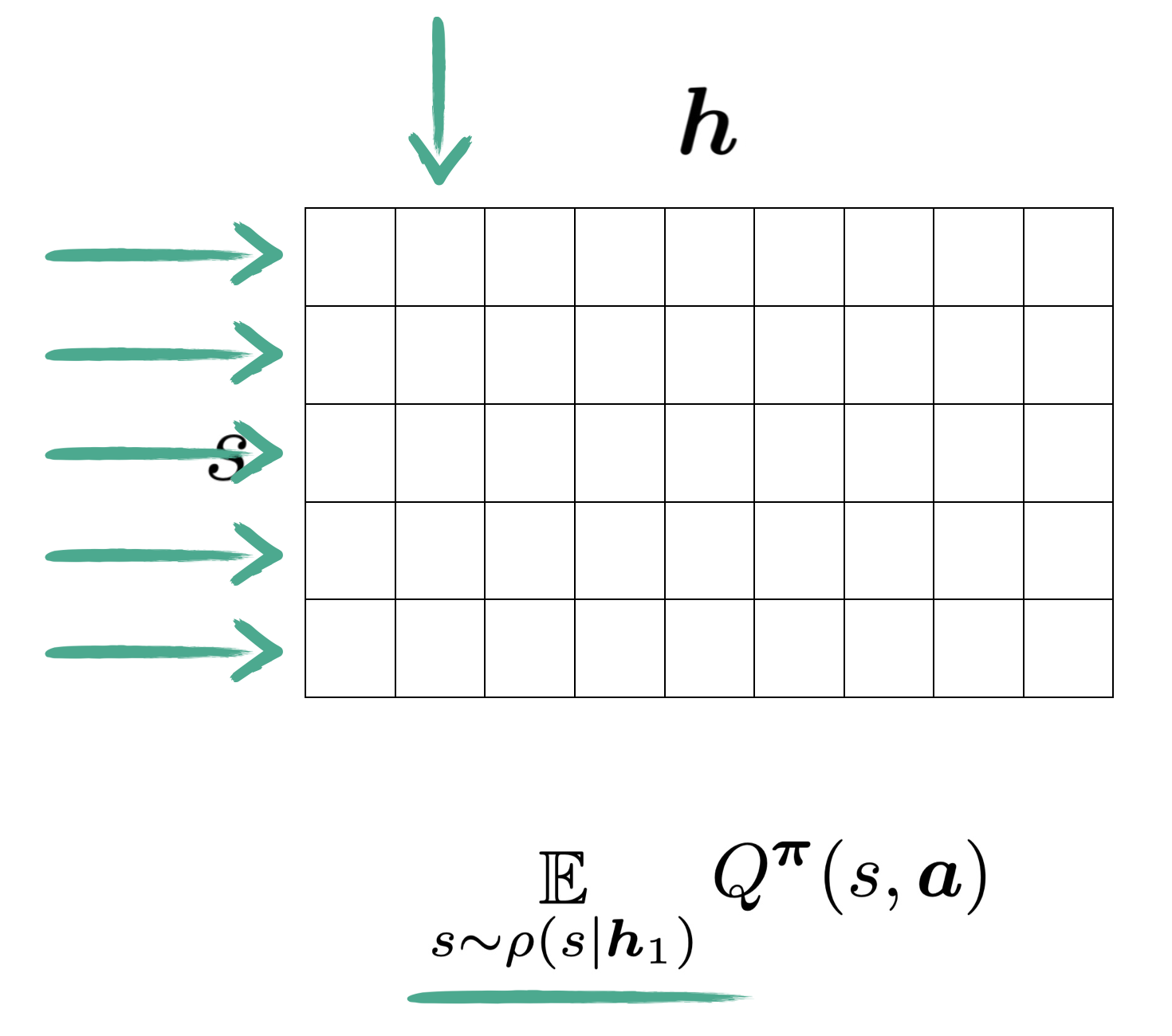So in essence, this value approximation for $\boldsymbol{h}_1$ becomes a weighted sum of the entire matrix.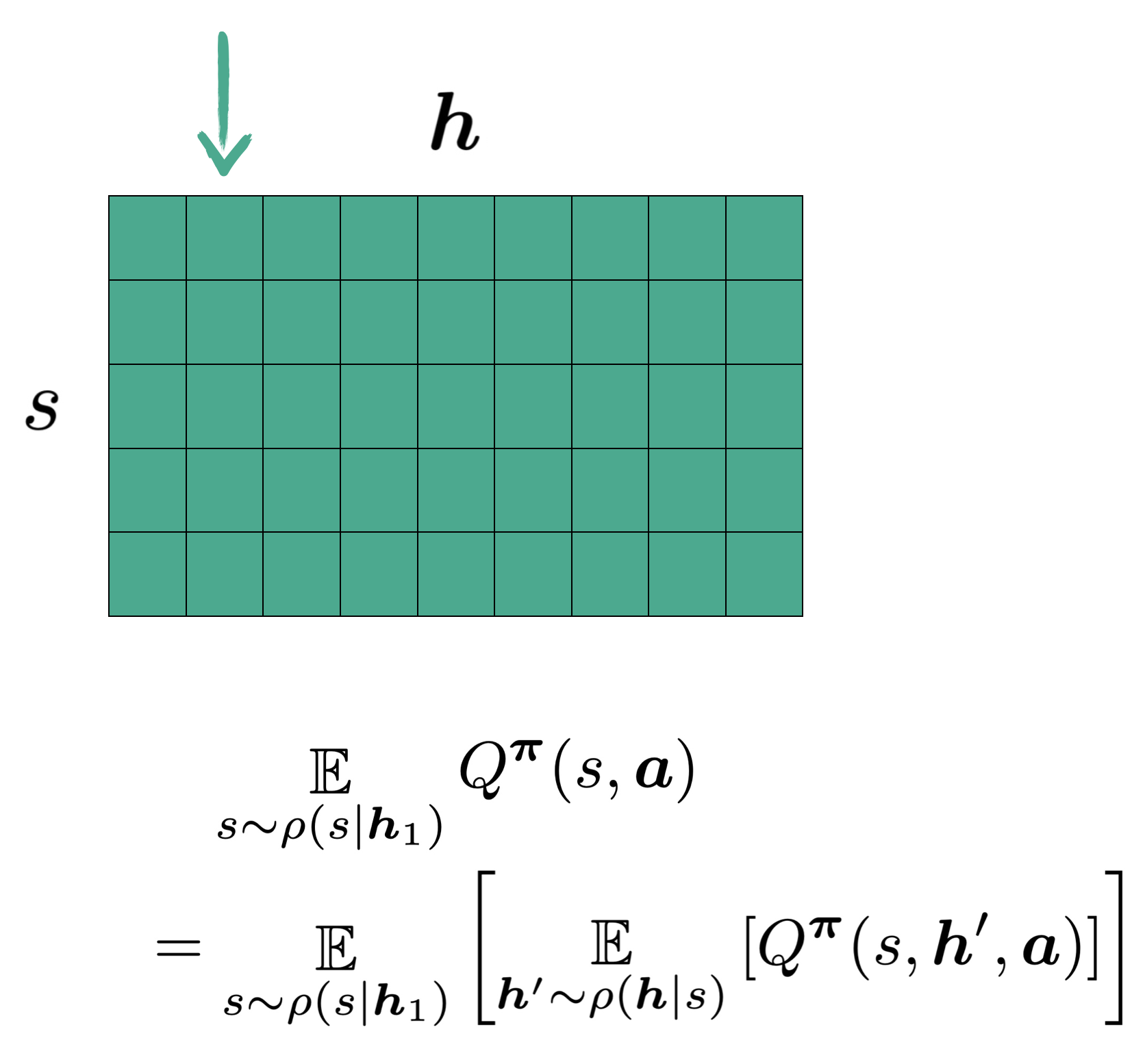As such, we conclude that using the state values to approximate history values is flawed.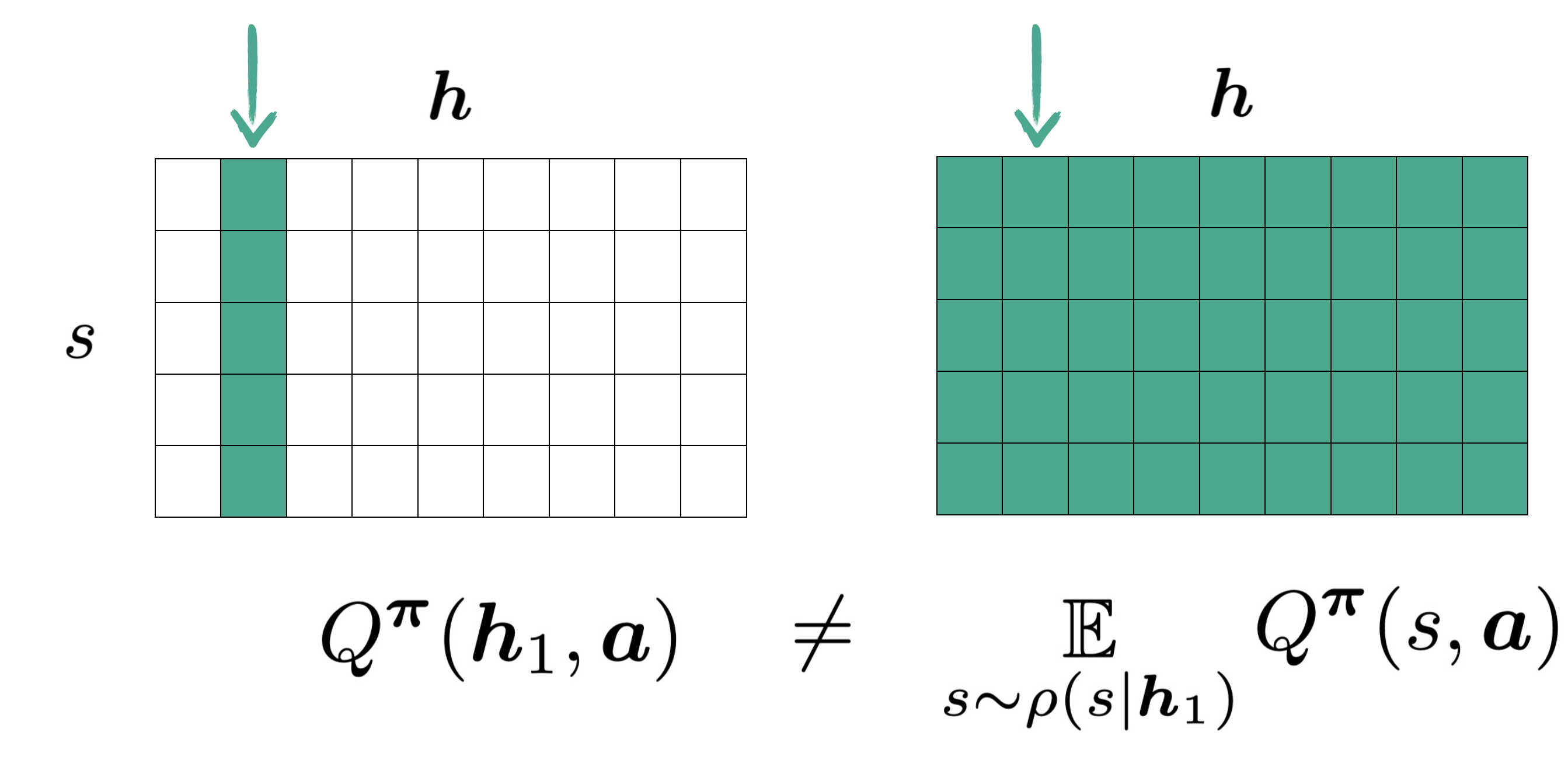There is a notable special case, though. Now think about it, for our approximation to be not biased, we would just need the yellow part to not interfere our mean (duh).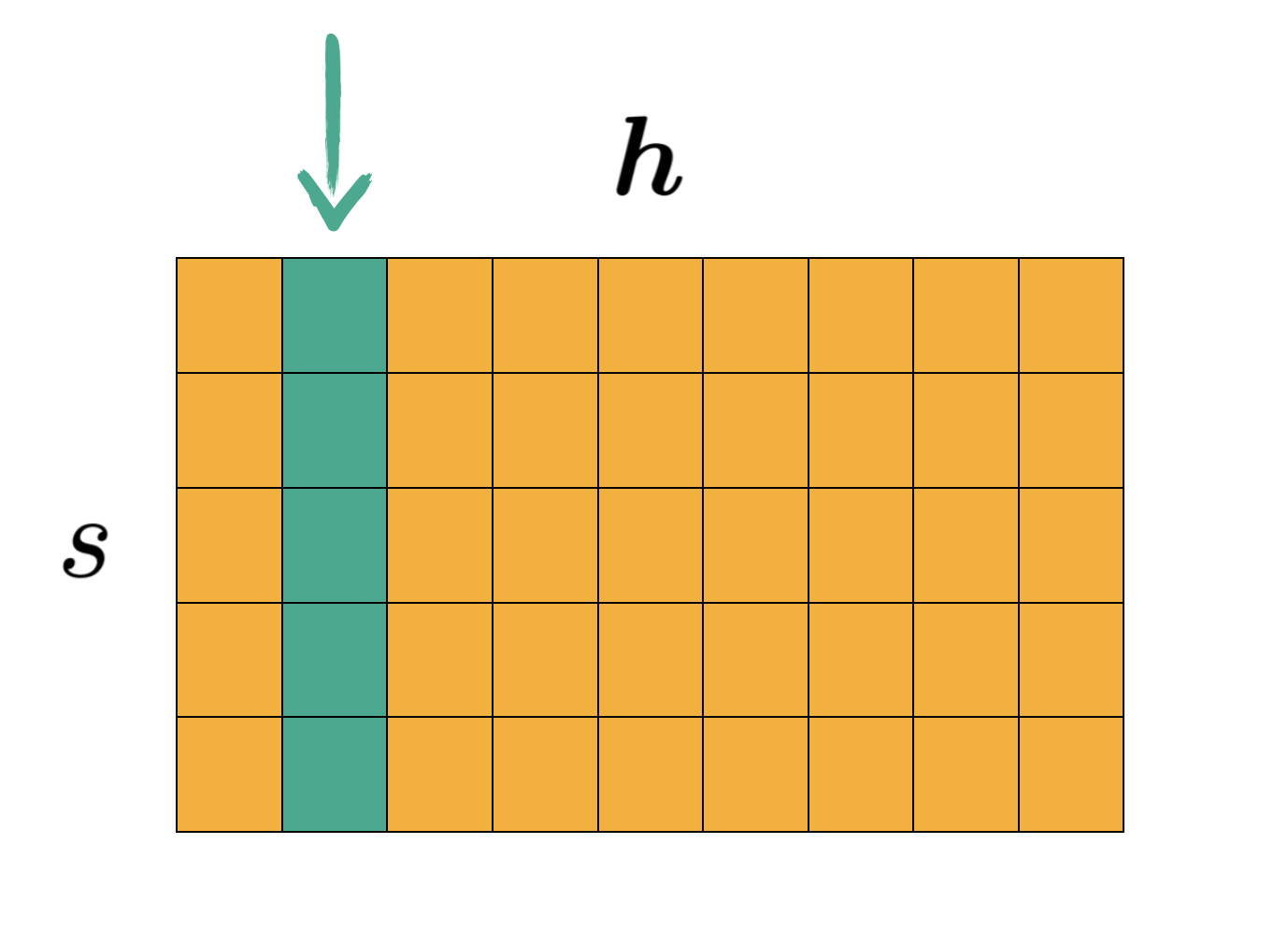So when would this happen? Errrrr… Every row have the same value? Yes, that should do it! And it so happens it is the case for having more information or less information does not matter from a value equivalent standpoint. And it is also the case with some benchmarks seen in the paper.